# How To Perform The Delta-Delta Ct Method

## What is the delta-delta Ct method?

The delta-delta Ct method, also known as the 2∆∆Ct method, is a simple formula used in order to calculate the relative fold gene expression of samples when performing real-time polymerase chain reaction (also known as qPCR). The method was devised by Kenneth Livak and Thomas Schmittgen in 2001 and has been cited over 61,000 times.

## Mastering qPCR

Further video tutorials on qPCR data analysis can be found in our Mastering qPCR course
>>Use code 20QPCR to get 20% off<<

## The FREE Microsoft Excel template

We have created a FREE Excel template which contains all of the formula described in this article below. Use this to practise and get the hang of the calculations. Everything is done for you, all that is required is the Ct values!

## Understanding the delta-delta Ct method formula

It is worthwhile understanding what the delta-delta Ct formula means before diving straight into the calculations.

The overall formula to calculate the relative fold gene expression level can be presented as:This looks like a scary mathematical formula when in actual fact, it isn’t. Let’s break the formula down into easier to understand chunks.

Firstly, Ct stands for the cycle threshold (Ct) of your sample. This is given after the qPCR reaction by the qPCR machine. Simply, it is the cycle number where the fluorescence generated by the PCR produce is distinguishable from the background noise.

The symbol refers to delta. Delta is a mathematical term used to describe the difference between two numbers. So it is useful to use when summarising long formulas.

So, let’s take a look to see what the ∆∆Ct part of the equation means:

∆∆Ct = ∆Ct (treated sample) – ∆Ct (untreated sample)

Essentially, ∆∆Ct is the difference between the ∆Ct values of the treated/experimental sample and the untreated/control sample. But what does ∆Ct refer to?

Let’s take a look:

∆Ct = Ct (gene of interest) – Ct (housekeeping gene)

Basically, ∆Ct is the difference in Ct values for your gene of interest and your housekeeping gene for a given sample. This is to essentially normalise the gene of interest to a gene which is not affected by your experiment, hence the housekeeping gene-term.

## Using the delta-delta Ct formula to calculate gene expression

To use the delta-delta Ct method, you require Ct values for your gene of interest and your housekeeping gene for both the treated and untreated samples. If you have more than one housekeeping gene, it may be worth checking out the guide on analysing qPCR data with numerous reference genes.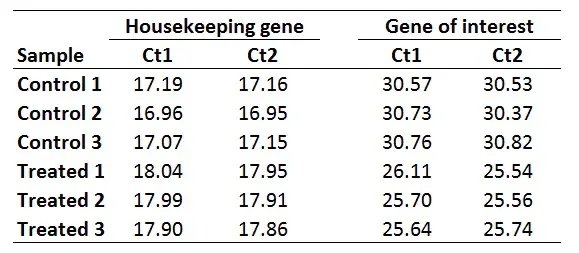Here is how to calculate the relative gene expression in 5 easy steps.

### 1. Average the Ct values for any technical replicates

The first step is to average the Ct values for the technical replicates of each sample. So, when performing the qPCR in duplicate or triplicate, for example, these values need to be averaged first. In the example below, each sample was run in duplicate (Ct1 and Ct2).

###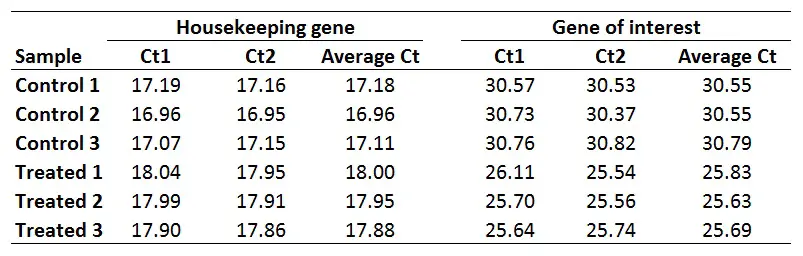2. Calculate the delta Ct for each sample

The next step is to calculate delta Ct (∆Ct) for each sample by using the newly created average Ct values. The formula to calculate delta Ct is presented below.

∆Ct = Ct (gene of interest) – Ct (housekeeping gene)

For example, to calculate the ∆Ct for the ‘Control 1‘ sample:

∆Ct Control 1 = 30.55 – 17.18

### 3. Select a calibrator/reference sample(s) to calculate delta delta Ct

The next step is to decide which sample, or group of samples, to use as a calibrator/reference when calculating the delta-delta Ct (∆∆Ct) values for all the samples. This is the part which confuses a lot of people. Basically, this all depends on your experiment set-up.

A common way of doing this is to just match the experimental samples and determine the relative gene expression ratios separately. This is all well and true for experiments that have matched pairs, such as the case in cell culture experiments. However, this is difficult when the two experimental groups vary in n numbers and do not have matched pairs.

Another way to select a calibrator/reference sample is to pick the sample with the highest Ct value, so the sample with the lowest gene expression. This way, all the results will be relative to this sample. Or, you could simply select just one of the control samples to act as the reference sample.

I personally average the ‘Average Ct’ values of the biological replicates of the control group to create a ‘Control average’. By doing so would mean that the results are presented relative to the control average Ct values.

Whichever sample, or group of samples, you use as your calibrator/reference is fine so long as this is consistent throughout the analyses and is reported in the results so it is clear. Remember, the results produced at the end are relative gene expression values.

With this in mind, if we want to get ∆∆Ct values for every sample (including for each control sample), we first need to average the ∆Ct for the 3 control samples:

∆Ct Control average = (13.38 + 13.60 + 13.68)/3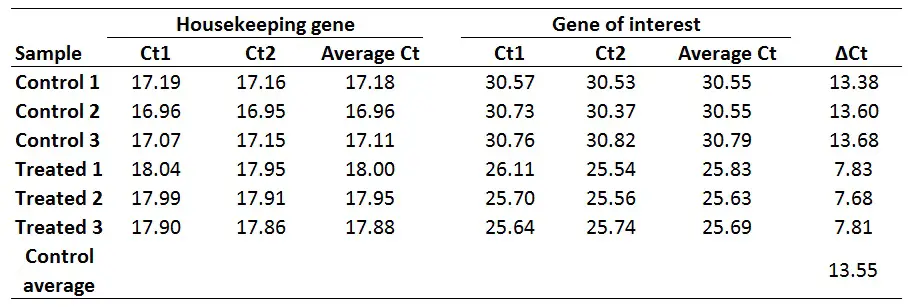### 4. Calculate delta delta Ct values for each sample

Now calculate the ∆∆Ct values for each sample. Remember, delta delta Ct values are relative to the untreated/control group in this example. The formula to calculate delta delta Ct is presented below.

∆∆Ct = ∆Ct (Sample) – ∆Ct (Control average)

For example, to calculate the ∆∆Ct for the Treated 1 sample:

∆∆Ct Treated 1 = 7.83 – 13.55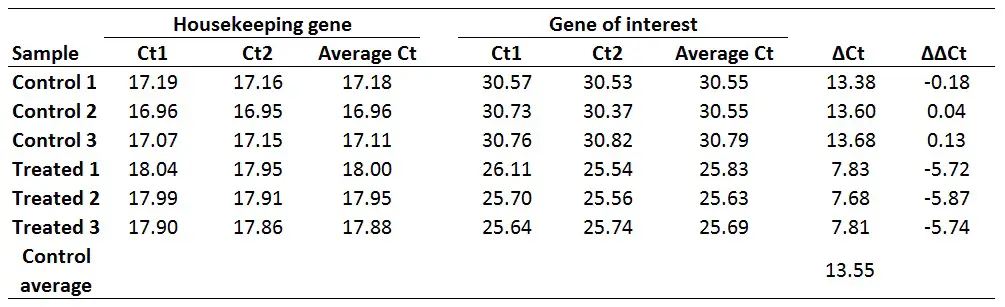### 5. Calculate the fold gene expression values

Finally, to work out the fold gene expression we need to do 2 to the power of negative ∆∆Ct (i.e. the values which have just been created). The formula for this can be found below.

Fold gene expression = 2^-(∆∆Ct)

For example, to calculate the fold gene expression for the Treated 1 sample:

Fold gene expression = 2^-(-5.72)

Doing this would give a fold gene expression of 52.71 for the Treated 1 sample. Doing this for all of the samples will look like this: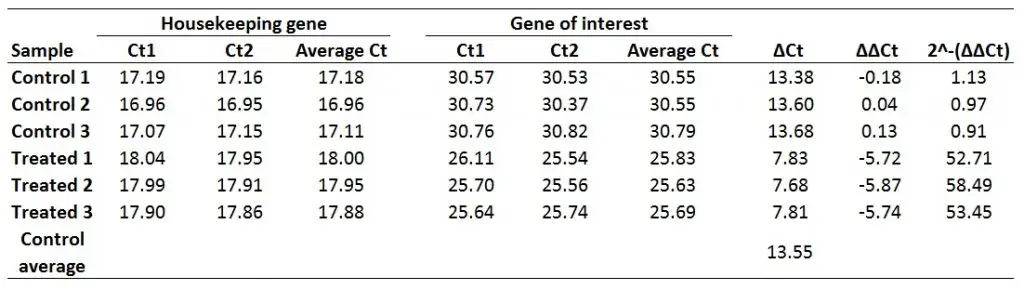And that is how you can use the delta-delta Ct method to work out the fold gene expression for your samples.

## Statistical analysis

Just a point regarding statistical analysis of the gene expression values. It is always best to log transform the values (2^-∆∆Ct) before undertaking statistical analysis. This is because the untransformed gene expression values will most likely not be normally distributed and heavily skewed, especially in experiments where a strong stimulus is used. To do log transformations in Excel, simply use the log formula (=Log).

Then, the choice of statistical test will be dependent on your experimental set-up. If you are struggling to perform a particular test, refer to our selection of SPSS and GraphPad Prism tutorials.

1.Shirin

Hi Steven,
Thank you so much for sharing that informative article.
I have a same question as Yogesh. How can I calculate or analyze gene expression data without control group and just based on Housekeeping gene? I have three housekeeping gene. Is it possible to just perform delta ct to get the ratio and then run Anova test based on mean of delta ct and variance? or I have to do 2 to the power of the delta ct value to get the relative gene expression and then perform statistics on?
Many thanks
Shirin

•Dr Steven Bradburn, PhD

Hi Shirin,
I would advise using the same approach as I recommended to Yogesh. Or use the method for analysing with multiple reference genes. Pick any sample in your experiment as the ‘calibrator’ since you do not have a control group/sample. Go right to the end and then log-transform your data before statistical analyses.
I hope that makes sense?
Steven

• Hi Steven,

Thank you very much. your answer was clear. Just wanted to double check is it fine if I just chose one or two samples with low gene expression as a calibrator?

Many thanks,
Shirin

2.Aurore

Hi Steven,

I have a question concerning the reference condition: my gene of interest is only expressed in my treated condition and not in my reference condition (Ct >40 or no value at all).
How can I do to calculate my deltadelta Ct ? If I use values >40 cycles, I obtain results that are not “correct” biologically.

•Aurore

Regards,
Aurore

•Dr Steven Bradburn, PhD

Hi Aurore,
In your case, you could just describe your results; stating that the reference condition was too low expressed to be detected (i.e. Ct >40). It sounds like the difference between your reference and treated groups are quite large anyway so you don’t need to perform statistical analyses to show this.
I hope that helps.
Steven

3.Ricardo Chiong

Thank you for sharing this. The gene of interest you used showed higher ct than the housekeeping gene, which I also observed in my previous experiments. But recently I did an qPCR which revealed a lower ct for my gene of interest in comparisson with the housekeeping (HMBS).My gene of interest is overexpressed in my samples (an oncogene in tumor), but I don’t know if that is suppose to happen. If I calculate the delta ct as ct [gene of interest] – ct [housekeeping gene] , I get a negative result. Is it correct to assume the delta ct would be just the difference between than, so it would be positive anyway? Is it the same for the delta delta ct formula (with negative results) if it’s a comparisson with the sample with lower expression?

•Dr Steven Bradburn, PhD

Hi Ricardo,
Usually your housekeeping gene should be strongly expressed (lower Ct value) compared with your gene of interest. But, in experiments where there is a strong stimulus then it is possible that the gene of interest can be more expressed. However, I would double-check your product, i.e. it is not primer-dimer (melt curve analysis or running product on a gel will answer this – if SYBR green assays are used), just to confirm there is nothing strange going on in the reaction.
The equations stay the same regardless of the Ct values, so the delta Ct is always the Ct[gene of interest]-Ct[housekeeping gene] – if that is what your last question refers to?
Thanks,
Steven

4.Maheshika

it was very useful! Thank you!

To calculate the standard deviation/error for error bars on the graph, which values would you use?

•Dr Steven Bradburn, PhD

Hi Maheshika,
Many thanks for the message.
For this I would calculate the standard error of the gene expression values at the end. You would need numerous biological replicates per group to be able to do this (i.e. repeat your experiment multiple times).
I hope that helps.
Steven

5.Yogesh Chander

Thanks for nice description on how to calculate ddCt. My question is how to calculate ddCt if you don’t have “Control” group? In my assay I have gene of interest and house keeping gene, is it still possible to calculate ddCt?

•Dr Steven Bradburn, PhD

Hi Yogesh,
Many thanks for your kind comment.
Sure it is possible. If you just have a group of samples instead of a control group you can just select 1 sample as the calibrator (control) sample. Then everything will be relative to this.
I hope that makes sense.
Steven

6.Marco

Hello Steven,
I used to do the analysis the same way you do, normalizing on the average of the controls. For the significance I usually perform the Pairwise Wilcoxon Rank Sum Tests.
However, I am now analyzing samples in which I have a lot of variability between biological replicates. If I normalize each replicate on it internal control this variability is greatly reduced. However, doing so the sample “control” becomes simply 1. What kind of non parametric test should I do to test whether the ddCT of my treatments are significantly different than 0? The Pairwise Wilcoxon Rank Sum Tests works with pair and I lose the control since it becomes = 1 in all the samples. Can I perform the one-sample Wilcoxon signed rank test with null hypothesis = 1 for each gene and then adjust for multiple comparison?
Thanks,
Marco

•Dr Steven Bradburn, PhD

Hi Marco,
I recommend log-transforming your gene expression data before performing statistical analyses. Either using the base of 10 or 2. This should help out with the normality of the data too. After doing this, would your data still be skewed or not normal? If it’s comparing 2 groups then either an independent student t-test or a Mann-Whitney U test should do the trick. Unless I am missing something? When selecting your calibrator sample you can select just one of the ‘controls’ that way only 1 sample in the experiment will have a 2^-ddCT of 1.
Thanks,
Steven

7.Fintan Mc Kenna

Hi Steven,

Really informative article. Your data is examining the changes in expression for control group and treated in GOI and HKG . I wanted to examine the effects that a non-coding variant in an enhancer sequence in one sample (called experimental) would have on gene expression in comparison to 3 healthy controls (control 1,2,3) in the GOI and GAPDH

My 2^-ΔΔCt values for control 1, 2, 3, are 0.687, 0.723 and 0.718. This would indicate that there is down regulation in the experimental sample in comparison to the 3 controls. My question is can statistical analysis be performed using the log transformation of these numbers ?

Fintan

•Dr Steven Bradburn, PhD

Hi Fintan,
It would be hard to perfrom statistical analysis if you have 1 sample in one group (experimental) and 3 samples in the other group (control). Ideally, you need more biological replicates, especially in your experimental group. Is there any way you can repeat the experiment?
Also regarding your set-up, which sample did you use as your ‘calibrator’ in the equation?
Many thanks,
Steven

8.KB

Following from my last response, how would I explain fold change when looking at the log(2^-ddCt) values? For example, the fold change for a sample was originally 0.7 fold increase (when the control 2^-ddCt = 1) but now the sample log value is 0.2 compared to the untreated log value of 0 – would I say it has 0.2 fold increase?

Thank you

•Dr Steven Bradburn, PhD

Hi Karolina,
I will e-mail you now.
Thanks,
Steven

9.Fatemeh

Hi Dr Bradburn, thanks for ur good explanations. When we test samples in duplicate, and between the duplicates is more than 1cycles differences, what would be the problem?
And how can I find out that the expression of my treated have enough change? What ddtc should I have that I could say my drug is infected?

•Dr Steven Bradburn, PhD

Hi Fatemeh,
If your technical replicates are >1 Ct apart then this could be errors in pipetting and handling. Try pipetting larger sample volumes into the reaction (eg 3 uL, as opposed to 0.5 uL). This will make it a lot easier.
For your second question, there is no magic answer. You will need to perform statistical tests (eg T tests) to determine any statistically signififcant differences between groups.
Many thanks,
Steven

10.Grace

Hi Steven..thank you for your great explanation. I am planning to do comparison of miRNA expression before and after treatment. in this case, is DDCT applicable? do I need a control group or the “before” treatment group will serve as control group it self?
I am a bit confused since many paper about miRNA expression mentioned only delta CT, not delta delta CT method..
Thanks

•Dr Steven Bradburn, PhD

Hi Grace,
You can use the DDCt method for miRNA only if you have a reference miRNA gene in your experiment. Many miRNA screening assays include these. You also need a control or before group, to act as the comparator. Alternatively, the delta Ct method is usually applied to miRNA analysis if there are no reference genes or specific experimental groups.
I hope that helps.
Best wishes,
Steven

11.KB

Hi Steven,

How would you obtain P-values and run statistical analysis even if you log the 2^-deltadeltaCt values as there is no 2^-deltadeltaCt value for the untreated alone? Really confused about how I can analyse the data besides obtaining 2^-deltadeltaCt and showing it in a graph.

Thank you 🙂

•Dr Steven Bradburn, PhD

Hi Karolina,
I advise log-transforming the 2^-DDCt values then performing your statistical analyses on these values. You control group will have an average value of 0, since the log of 1 is 0.
I hope that makes sense.
Best wishes,
Steven

•KB

Thank you for your response! Would it be best to use the transformation data to display in a graph or is it possible to use the original values and indicate which values are significant based on the analyses from the transformed data?

Thank you 🙂

•Dr Steven Bradburn, PhD

Hi Karolina,
You could do either. To save confusion I would plot and perform the statistical analyses using the transformed data.
Best wishes,
Steven

12.mona

dear steven ,
thanks for ur quick response
ddct was 2.35

•Dr Steven Bradburn, PhD

Hi Mona,
If the ddtc was 2.35, then the 2^-ddct will be 2^(-2.35) which is 0.196.
Thanks,
Steven

13.mona

hi steven, thanks alot for ur incredible explanation , my 2^-ddct is -0.3 what is that mean ?

•Dr Steven Bradburn, PhD

Hi Mona,
What was the ddCt value for this sample (the step before the final 2^-ddct)?
Thanks,
Steven

14.Zachary Visser

Hi Steven,

I am just wondering how I would go about calculating standard error, or similarly a 95% confidence interval using the related standard deviations using this method.

Thanks,

Zach

•Dr Steven Bradburn, PhD

Hi Zach,
Are you asking how to calculate the SE from SD?
Thanks,
Steven

15.gizem

Hi Steven
Thank you for a great text and explanation of a method.
ı have three groups: malignant tumor- corresponding non-neoplastic tissue and another benign disease group
I am wondering, Can ı use corresponding non-neoplastic tissues average delta CT value to calculate benign disease group delta delta CT value

•Dr Steven Bradburn, PhD

Hi Gizem,

Yes, you can use the non-neoplastic tissue group as the ‘calibrator sample/group’. When reporting the results, you will have to stress that the results are relative to the non-nepplastic group.

I hope that makes sense,

Steven